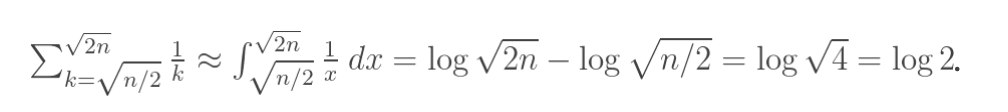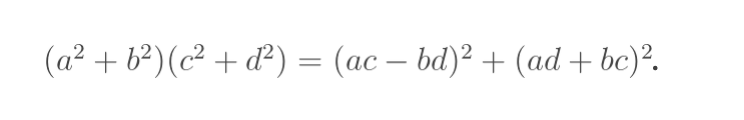This piece was originally published on God Plays Dice.

Patrick Honner tweeted a few days ago:

So let’s say that a number is a “puzzle number” if it’s of the form m x n with m ≤ n ≤ 2m — that is, a puzzle has to have an aspect ratio between 1 (square) and 2. (The choice of 2 is arbitrary here, but any other constant would be more arbitrary.) We can easily work out the first few puzzle numbers:

2 = 1×2, 4 = 2×2, 6 = 2×3, 8 = 2×4, 9 = 3×3

which is enough to find them in the OEIS: that’s A071562, defined as “Numbers n such that the sum of the middle divisors of n (A071090) is not zero.” (What’s a “middle divisor”, you ask? It’s a divisor of n that’s between (square root n/2) and (square root 2n.)) Titling it that way seems a bit strange to me: I’d have called it “Numbers such that the number of middle divisors of n (A067742) is nonzero.”

The first 10,000 puzzle numbers have been calculated; the 10,000th is 35,956. There are 43 under 100, 336 under 1,000, and 2,932 under 10,000 — it doesn’t appear that they have constant density. It’s not hard to take this further — there are 26,870 under 10^5, 252,756 under 10^6, 2,409,731 under 10^7 and 23,169,860 under 10^8. For example, in R, you can generate the puzzle numbers as follows. (This take a few seconds to run. Note that you don’t have to compute any prime factorizations.)

N = 10^8
= rep(0, N)

for (m in 1:floor(sqrt(N))){
_max_n = min(2m,floor(N/m))_
_a[m
(m:max_n)] = a[m*(m:max_n)]+1_
}

puzzle = which(a >= 1)

I had at first thought this sequence had a natural density, because I was only trying numbers up to a few hundred in my head, and because the number of middle divisors appears to average somewhere around log (2). There’s a nice heuristic for this — a middle divisor of n is somewhere between (square root n/2) and (square root 2n); the “probability” that a number is divisible by k is 1/k, so the “expected number” of middle divisors of n is:But there must be more numbers as you go further out that have many middle divisors, and more zeroes. This is similar to the behavior you see for the problem of “how many ways can an integer be written as a sum of two squares.” In that case the “average” is π/4**,** but an integer greater than one can be written as a sum of two squares if and only if its prime decomposition contains no prime congruent to 3 mod 4 raised to an odd power; asymptotically the number of positive integers below x which are the sum of two squares goes like bx / (square root log x) for a constant b (the constant is the Landau-Ramanujan constant). That connection might stem from the fact that both sequences are multiplicative. For sums of two squares, that follows from the factorization-based characterization or from the fact thatFor puzzle numbers, it follows from a remark of Franklin T. Adams-Watters in the OEIS entry. So numbers which have lots of factors both tend to be sums of two squares and to be puzzle numbers in many different ways; as we get to bigger numbers those start “crowding out” the smaller numbers.

The math internet has noticed this at least once before. John D. Cook wrote in 2014: “Jigsaw puzzles that say they have 1,000 pieces have approximately 1,000 pieces, but probably not exactly 1,000. Jigsaw puzzle pieces are typically arranged in a grid, so the number of pieces along a side has to be a divisor of the total number of pieces. This means there aren’t very many ways to make a puzzle with exactly 1,000 pieces, and most have awkward aspect ratios.”

1000 as 25-by-40 seems reasonable, but 27 x 37 = 999 or 28 x 36 = 1008 would also work. I assume that you wouldn’t actually see a 999-piece puzzle because the lawyers would claim calling that 1000 was false advertising. A puzzle blog indicates that most “500-piece” puzzles are actually 27 x 19 = 513 and most “1000-piece” puzzles are 38 x 27 = 1026 – both of these aspect ratios are approximations of (square root 2). That is a good aspect ratio to use if you want the ability to make both “500-piece” and “1000-piece” (or more generally, “N-piece” and “2N-piece”) versions of the same puzzle. Similarly the A0, A1, … series of paper sizes used in most of the world have that aspect ratio and therefore an An piece of paper can be cut into two A(n+1) pieces.

This post has been read 44 times!

0 0 vote
Article Rating

#### You Might Also LikeNot Optional
Optional